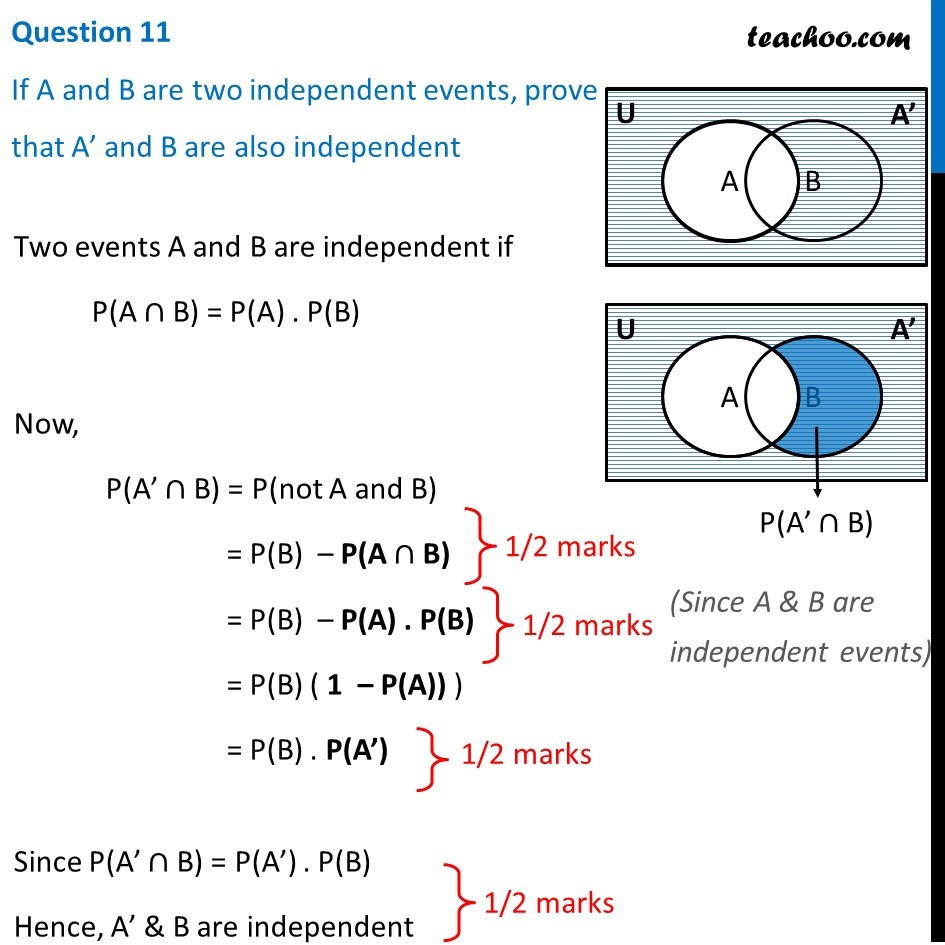CBSE Class 12 Sample Paper for 2019 Boards

Class 12
Solutions of Sample Papers and Past Year Papers - for Class 12 Boards

Question 11

If A and B are two independent events, prove that A’ and B are also independentLearn in your speed, with individual attention - Teachoo Maths 1-on-1 Class

### Transcript

Question 11 If A and B are two independent events, prove that A’ and B are also independent Two events A and B are independent if P(A ∩ B) = P(A) . P(B) Now, P(A’ ∩ B) = P(not A and B) (Since A & B are independent events) = P(B) – P(A ∩ B) = P(B) – P(A) . P(B) = P(B) ( 1 – P(A)) ) = P(B) . P(A’) Since P(A’ ∩ B) = P(A’) . P(B) Hence, A’ & B are independent